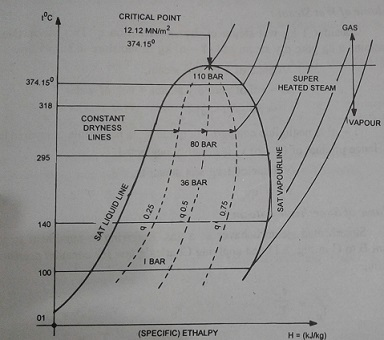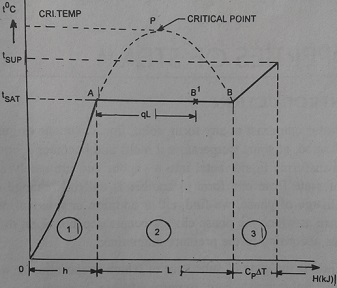TEMPERATURE - ENTHALPY DIAGRAM (T-H CHART):

Figure shows temperature - enthalpy graph drawn for a wide range of pressures. If all the liquid enthalpy (sensible heat) points obtained at different temperatures are joined we get 'saturated liquid line'. If all the dry saturated steam points are joined by means of a smooth curve we get a `saturated vapour line'. This line and the saturated liquid line enclose an area in which the steam is wet. To the left of saturated liquid line state of fluid is water. To the right to saturated vapour line steam is in superheated form. The wet steam area has constant dryness line drawn in it as shown dotted. Points lying along such a line have same dryness fraction.

The saturated liquid line and the saturated vapour line when continued upward eventually meet at a common point called 'critical point'. This point occurs at a pressure of 221.2 bar (22.12 MN/m^2) and a temperature of 374.15° C. It will be noticed that as the pressure increases towards the critical pressure, the enthalpy of evaporation (latent heat) reduces until it fmally becomes zero at the critical point. This implies that at the critical point the water changes directly into dry saturated steam.The critical point is the division between the behavior of fluid as a vapour and as a gas. At temperature higher than the critical temperature the vapour becomes a gas.

ENTHALPY FORMULAE :

Considering a unit mass of fluid (m=1 kg) we arrive at following calculations. Refer to Fig.Liquid enthalpy or sensible heat = h kJ/kg

Enthalpy of dry saturated steam

Hs = Liquid enthalpy + Enthalpy of evaporation

i.e., Hs = h + L kJ/kg.

Enthalpy of dry saturated steam is the quantity of heat required to convert 1 kg water at 0°C into dry saturated steam at constant pressure.

Enthalpy of wet steam, H's = h+ qL kJ/kg

Enthalpy of superheated steam,

Hsup = Enthalpy of dry sat steam + superheat enthalpy

i.e. Hsup = Hs + Cp ΔT

where ΔT = degree of superheat = superheat steam temperature, — saturation temperature.

= (tsup— tsat)° K

Being a constant pressure heating process, the superheat enthalpy = mCp ΔT kJ and

specific enthalpy = Cp ΔT kJ/kg.

Cp = mean value of specific heat of steam at constant pressure = 2.0934 kJ/kg K.